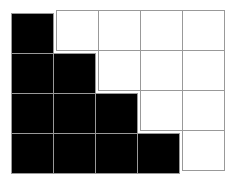# How To Compute 1+2+…+n and How to Remember How

I was recently writing a test for an object containing a map of currency amounts for months by month number. I needed to verify that each value was exposed through a month-specific attribute and that it exposed the sum of values across all the months through another attribute.

For test data, I chose the following map:

Obviously, those 12 thousands add up to 12,000, but what is the contribution of those 1–to–12 parts? I could have just added the numbers together, but there’s an easier way.

That easier way is the formula 12 ⨉ 13 / 2 or more generally, n ⨉ (n + 1) / 2, and in the case above, that’s 78, but how do I remember that formula? This is something I need to know somewhat often, but not quite often enough to simply have the formula memorized. The way that I remember how to re–create this formula quickly when I need it is with a nifty visual mnemonic that I’d like to share. For this example, we’ll say that n is equal to 4.The black squares (the ones we count) form a triangle, and the white squares (the ones we don’t count) form an identical triangle rotated 180 degrees. If we stick those triangles together as shown, we get a 4 x 5 rectangle, and since each triangle in the rectangle has the same number of squares, that means half of the squares in the rectangle are black.